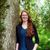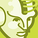# QlikView App Dev

Discussion Board for collaboration related to QlikView App Development.

Announcements
Join this live chat April 6, 10AM EST - QlikView to Qlik Sense REGISTER
cancel
Showing results for
Search instead for
Did you mean:Not applicable

## calculated dimension

why cannot i do this in a calculated dimension?

=if(sum({<DateCleanLog={"=\$(=Date(Today()-1))"}>} Rows) = 0, null(), LogCustomerID)

so if there was no rows yesterday, then dont show logcustomerid, else show customerid  - why cnat i do so?

1 Solution

Accepted SolutionsMVP

Sum is an aggregation expression, and in order to use it in a dimension, it must be used within aggr(), so something like the following may work for you:

=aggr(if(sum({<DateCleanLog={"=\$(=Date(Today()-1))"}>} Rows)= 0, null(), LogCustomerID), LogCustomerID)

3 RepliesMVP

Sum is an aggregation expression, and in order to use it in a dimension, it must be used within aggr(), so something like the following may work for you:

=aggr(if(sum({<DateCleanLog={"=\$(=Date(Today()-1))"}>} Rows)= 0, null(), LogCustomerID), LogCustomerID)MVP

Because when you perform the sum, the same sum is computed according specified dimensions so if you need something like the one you have written you must use Aggr function

Hope it helpsChampion

beacuse aggregate function we can use directly in dimension

it will show you "Error in dimension"

so for your expression to work you need to use aggr keyword available in qlikview

if(aggr(sum({<DateCleanLog={"=\$(=Date(Today()-1))"}>} Rows),LogCustomerID) = 0 ,null(), LogCustomerID)

then it whould work

basically aggr function used to group by some field  as is in ur example i have grouped your  expression of sum by

LogCustomerID

hope this helps